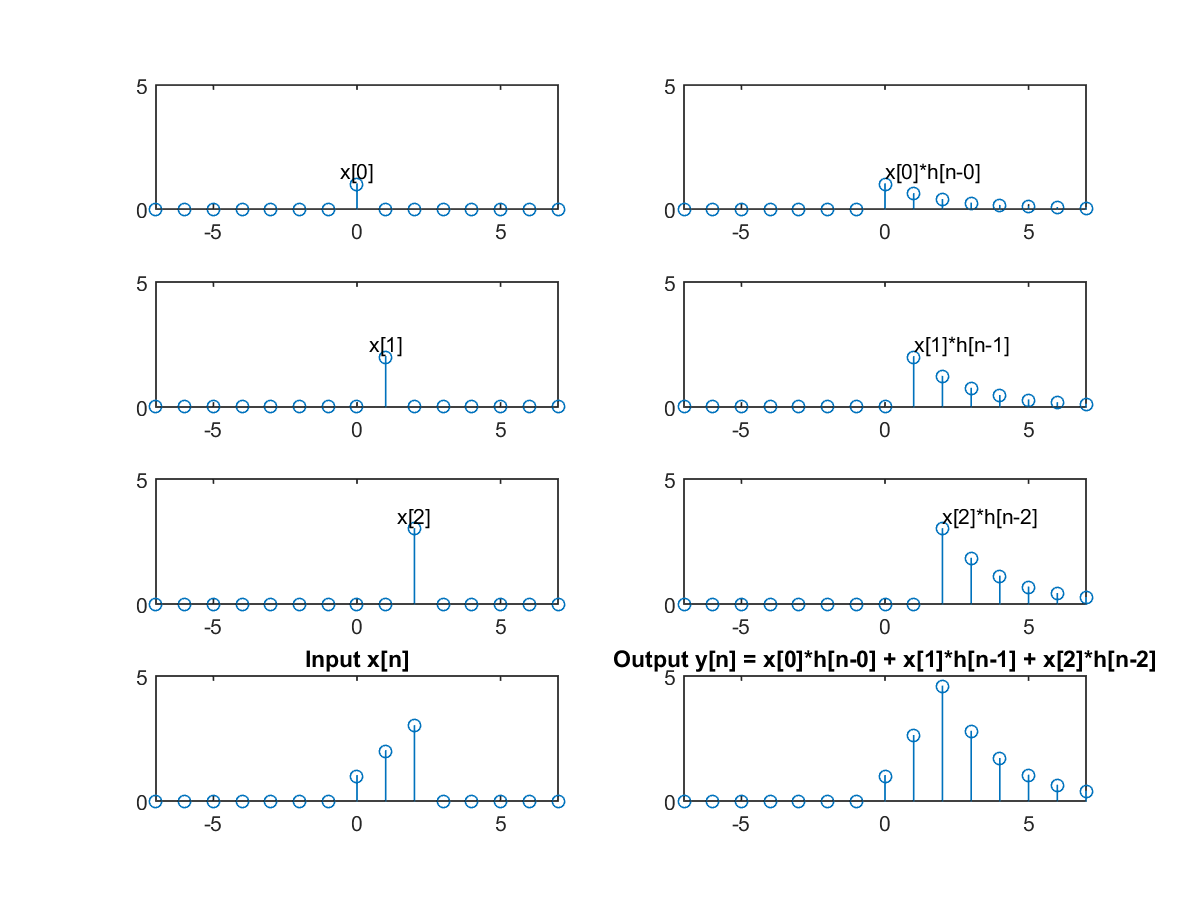## 9-3 Convolution (嚙踝蕭嚙踝蕭嚙踝蕭)

For any given arbitrary signal x[n], we can express it as a linear combination of the unit impulse signals, as follows:

x[n] = Sk=0x[k]d[n-k]
The right-hand side of the above equation can be viewed as the situation where
• k is the index for time.
• x[k] is a fixed function of time index k.
• d[n-k] is a function parameterized by n.
Similarly, the above equation can be rewritten into another format:
x[n] = Sk=0x[n-k]d[k]
The right-hand side of the above equation can be viewed as the situation where
• k is the index for time.
• d[k] is a fixed function of time index k.
• x[n-k] is a function parameterized by n.

For a given LTI system L{•}, when the input signal x[n] is decomposed by the first method, the output y[n] can be expressed as follows:
 y[n] = L{x[n]} = L{Sk=0∞x[k]d[n-k]} = Sk=0∞x[k]L{d[n-k]} = Sk=0∞x[k]h[n-k]
where h(n-k) = L{d(n-k)} is the response of the system with respect to the input of the unit impulse signal at n = k. In other words, the output of an LTI system is determined by the input signal x[n] and the system's impulse response h[n]. More specifically, the impulse response of an LTI system exclusively determine the characteristics of the system.

We can use the following plots to demonstrate the operations of the above formula:

Example 1: convolution01.m% Plot the operation of convolution n = -7:7; x = [0 0 0 0 0 0 0 1 2 3 0 0 0 0 0]; subplot(4,2,7); stem(n, x); limit=[min(n), max(n), 0, 5]; axis(limit); title('Input x[n]'); subplot(4,2,1); x0=0*x; x0(8)=x(8); stem(n, x0); axis(limit); h=text(0, x0(8), 'x'); set(h, 'horiz', 'center', 'vertical', 'bottom'); subplot(4,2,2); y0=0*x; index=find(x0); for i=index:length(n) y0(i)=x0(index)*exp(-(i-index)/2); end stem(n, y0); axis(limit); h=text(0, x0(8), 'x*h[n-0]'); set(h, 'vertical', 'bottom'); subplot(4,2,3); x1=0*x; x1(9)=x(9); stem(n, x1); axis(limit); h=text(1, x1(9), 'x'); set(h, 'horiz', 'center', 'vertical', 'bottom'); subplot(4,2,4); y1=0*x; index=find(x1); for i=index:length(n) y1(i)=x1(index)*exp(-(i-index)/2); end stem(n, y1); axis(limit); h=text(1, x1(9), 'x*h[n-1]'); set(h, 'vertical', 'bottom'); subplot(4,2,5); x2=0*x; x2(10)=x(10); stem(n, x2); axis(limit); h=text(2, x2(10), 'x'); set(h, 'horiz', 'center', 'vertical', 'bottom'); subplot(4,2,6); y2=0*x; index=find(x2); for i=index:length(n) y2(i)=x2(index)*exp(-(i-index)/2); end stem(n, y2); axis(limit); h=text(2, x2(10), 'x*h[n-2]'); set(h, 'vertical', 'bottom'); subplot(4,2,8); stem(n, y0+y1+y2); axis(limit); title('Output y[n] = x*h[n-0] + x*h[n-1] + x*h[n-2]');If we choose to use the second method for decomposing x[n], then y[n] can be expressed as follows:
 y[n] = L{x[n]} = L{Sk=0∞x[n-k]d[k]} = Sk=0∞x[n-k]L{d[k]} = Sk=0∞x[n-k]h[k]}

Since the computation of y[n] is used frequently, we shall define the convolution of two signals x[n] and h[n] as follows: y[n] = x[n]＊h[n] = Sk=0x[k]h[n-k]} = Sk=0x[n-k]h[k]}
The convolution operator has the following characteristics:

1. Commutative law: x[n]＊y[n] = y[n]＊x[n]
2. Associative law: (x[n]＊y[n])＊z[n] = x[n]＊(y[n]＊z[n])
3. Distributive law: x[n]＊(y[n]+z[n]) = x[n]＊y[n]+x[n]＊z[n]
4. Shift property: y[n]=x[n]＊h[n] → y[n-n1-n2]=x[n-n1]＊h[n-n2]
5. Convolving with unit impulse: x[n]＊d[n] = x[n]
6. Width: If duration(x[n])=N1 and duration(y[n])=N2, then duration(x[n]＊y[n])=N1+N2-1.

Audio Signal Processing and Recognition (音訊處理與辨識)